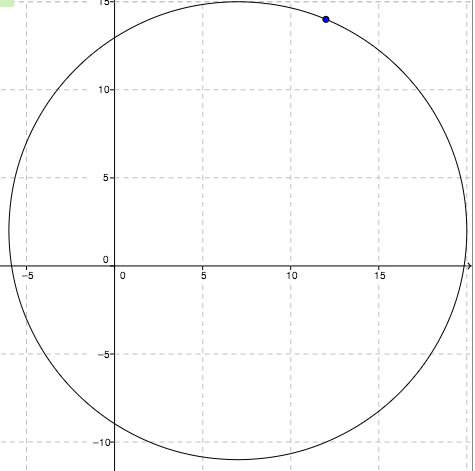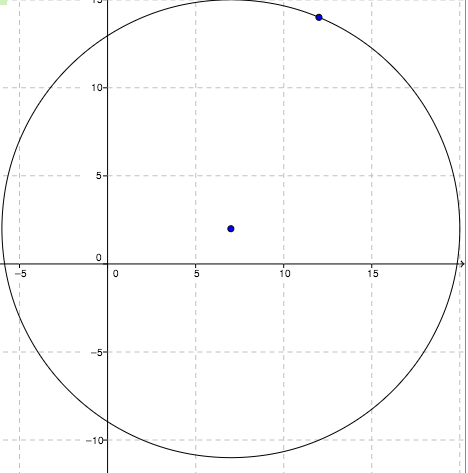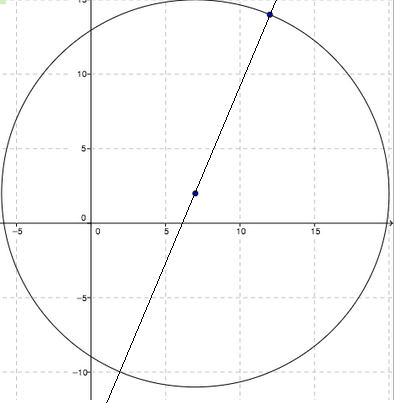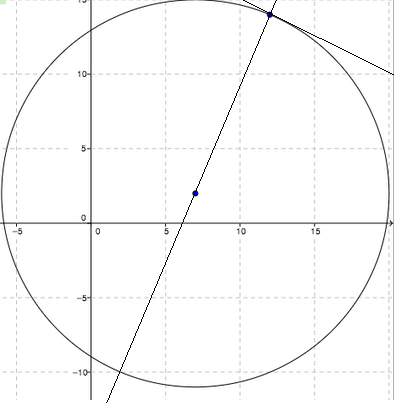### Home > A2C > Chapter Ch11 > Lesson 11.2.4 > Problem11-91

11-91.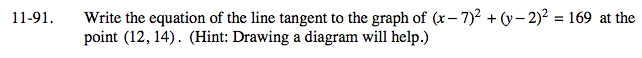Draw a diagram to show the situation.

Find the center of the circle. C:(7, 2)

Now find the line passing through the center and given point.

$\textit{y}=-\frac{12}{5}\textit{x}-14.8$

Now find the perpendicular line.

$\textit{y}=-\frac{5}{12}\textit{x}+19$